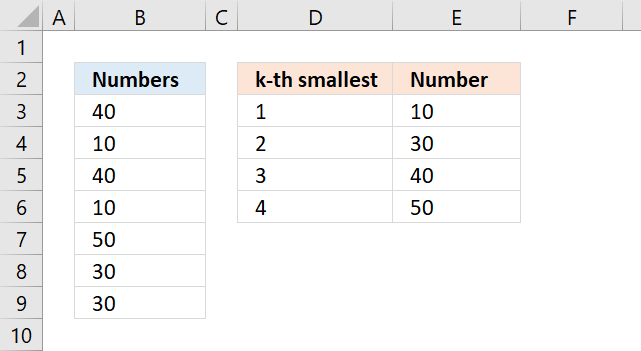Author: Oscar Cronquist Article last updated on December 12, 2017The formulas in column E, shown in the picture above, extracts the k-th smallest value from B3:B9 ignoring the duplicate numbers.

The following formula in cell E3 extracts the smallest number from B3:B9.

=MIN(B3:B9)

However, we need to use another formula in the cells below to ignore duplicate values. The formula in cell E4 extracts the second smallest number from B3:B9.

When you copy this formula and paste to cells below it will extract third, fourth, and so on, smallest value ignoring duplicate values.

=MINIFS(\$B\$3:\$B\$9, \$B\$3:\$B\$9, ">"&E3)

The MINIFS function returns the smallest value depending on the condition, in this case, it looks for values larger than the previous value in the cell above, meaning it will ignore duplicate numbers.

I can't use this formula in cell E3 because there is no formula above it.

The MINIFS function was introduced in Excel 2016, if you have an earlier version of Excel see example below.Formula in cell E3:

=MIN(B3:B9)

Array formula in cell E4:

=MIN(IF(E3<\$B\$3:\$B\$9, \$B\$3:\$B\$9, ""))

The formula above is an array formula. To enter an array formula, type the formula in a cell then press and hold CTRL + SHIFT simultaneously, now press Enter once. Release all keys.

The formula bar now shows the formula enclosed with curly brackets telling you that you entered the formula successfully. Don't enter the curly brackets yourself.